# How to Calculate and Solve for Turbine Stiffness (Correction Factor) | Irrigation Water Requirement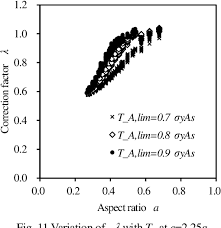The image above represents turbine stiffness.

To compute for turbine stiffness (correction factor), two essential parameters are needed and these parameters are Correction Factor for Radius of Curvature with Deflection (c) and Parallel Plate Stiffness for Tubing (σp).

The formula for calculating turbine stiffness:

σ = 0.149c[σp]

Where:

σ = Turbine Stiffness
c = Correction Factor for Radius of Curvature with Deflection
σp = Parallel Plate Stiffness for Tubing

Let’s solve an example;
Find the turbine stiffness when the correction factor for radius of curvature with deflection is 15 and the parallel plate stiffness for tubing is 3.

This implies that;

c = Correction Factor for Radius of Curvature with Deflection = 15
σp = Parallel Plate Stiffness for Tubing = 3

σ = 0.149c[σp]
σ = 0.149(15)
σ = 6.705

Therefore, the turbine stiffness is 6.705.

Calculating for the Correction Factor for Radius of Curvature with Deflection when the Turbine Stiffness and the Parallel Plate Stiffness for Tubing is Given.

c = σ / 0.149 x σp

Where;

c = Correction Factor for Radius of Curvature with Deflection
σ = Turbine Stiffness
σp = Parallel Plate Stiffness for Tubing

Let’s solve an example;
Find the correction factor for radius of curvature with deflection when the turbine stiffness is 32 and the parallel plate stiffness for tubing is 8.

This implies that;

σ = Turbine Stiffness = 32
σp = Parallel Plate Stiffness for Tubing = 8

c = σ / 0.149 x σp
c = 32 / 0.149 x 8
c = 32 / 1.192
c = 26.84

Therefore, the correction factor for radius of curvature with deflection is 26.84.

Calculating for the Parallel Plate Stiffness for Tubing when the Turbine Stiffness and the Correction Factor for Radius of Curvature with Deflection is Given.

σp = σ / 0.149 x c

Where;

σp = Parallel Plate Stiffness for Tubing
σ = Turbine Stiffness
c = Correction Factor for Radius of Curvature with Deflection

Let’s solve an example;
Find the parallel plate stiffness for tubing when turbine stiffness is 40 and the correction factor for radius of curvature with deflection is 6.

This implies that;

σ = Turbine Stiffness = 40
c = Correction Factor for Radius of Curvature with Deflection = 6

σp = σ / 0.149 x c
σp = 40 / 0.149 x 6
σp = 40 / 0.894
σp = 44.74

Therefore, the parallel plate stiffness for tubing is 44.74.

Nickzom Calculator – The Calculator Encyclopedia is capable of calculating the turbine stiffness.

To get the answer and workings of the turbine stiffness using the Nickzom Calculator – The Calculator Encyclopedia. First, you need to obtain the app.

You can get this app via any of these means:

To get access to the professional version via web, you need to register and subscribe for NGN 2,000 per annum to have utter access to all functionalities.
You can also try the demo version via https://www.nickzom.org/calculator

Apple (Paid) – https://itunes.apple.com/us/app/nickzom-calculator/id1331162702?mt=8
Once, you have obtained the calculator encyclopedia app, proceed to the Calculator Map, then click on Agricultural under Engineering.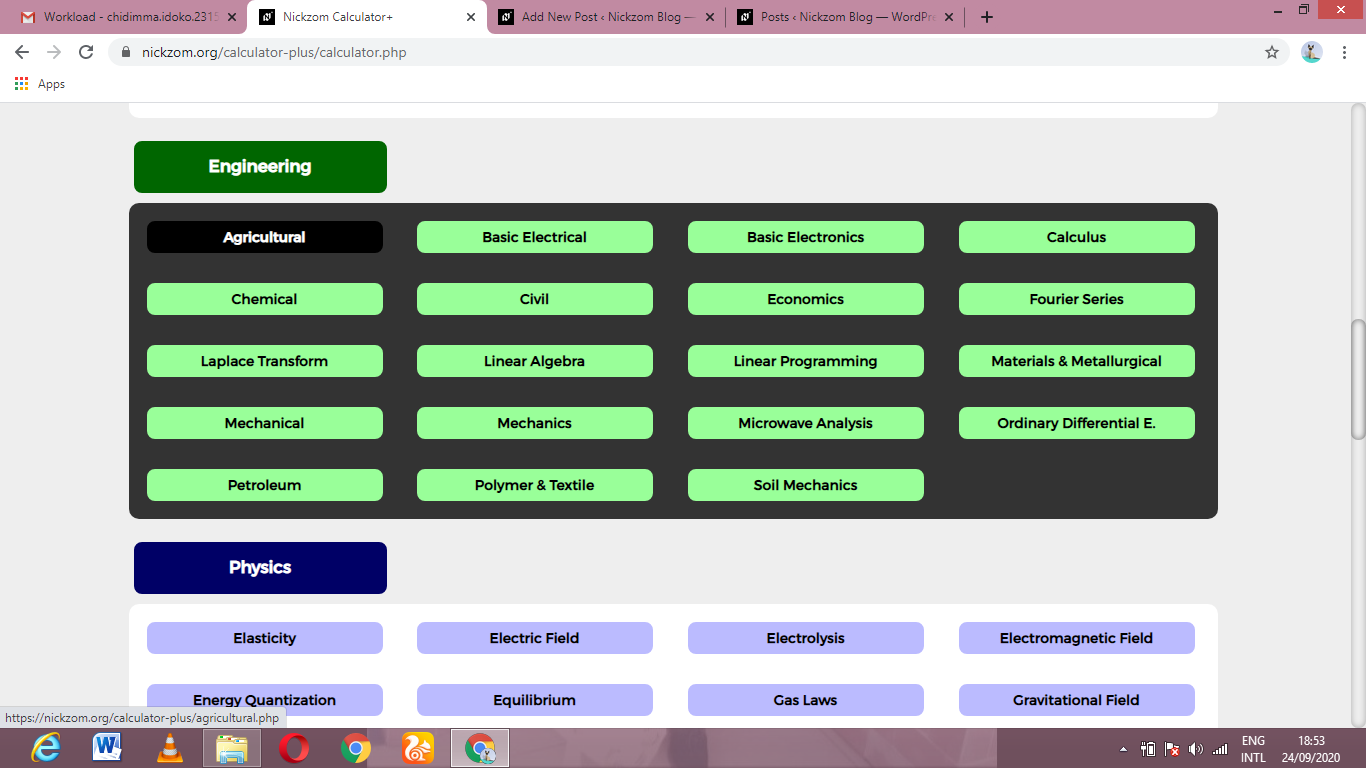Now, Click on Irrigation Water Requirement under Agricultural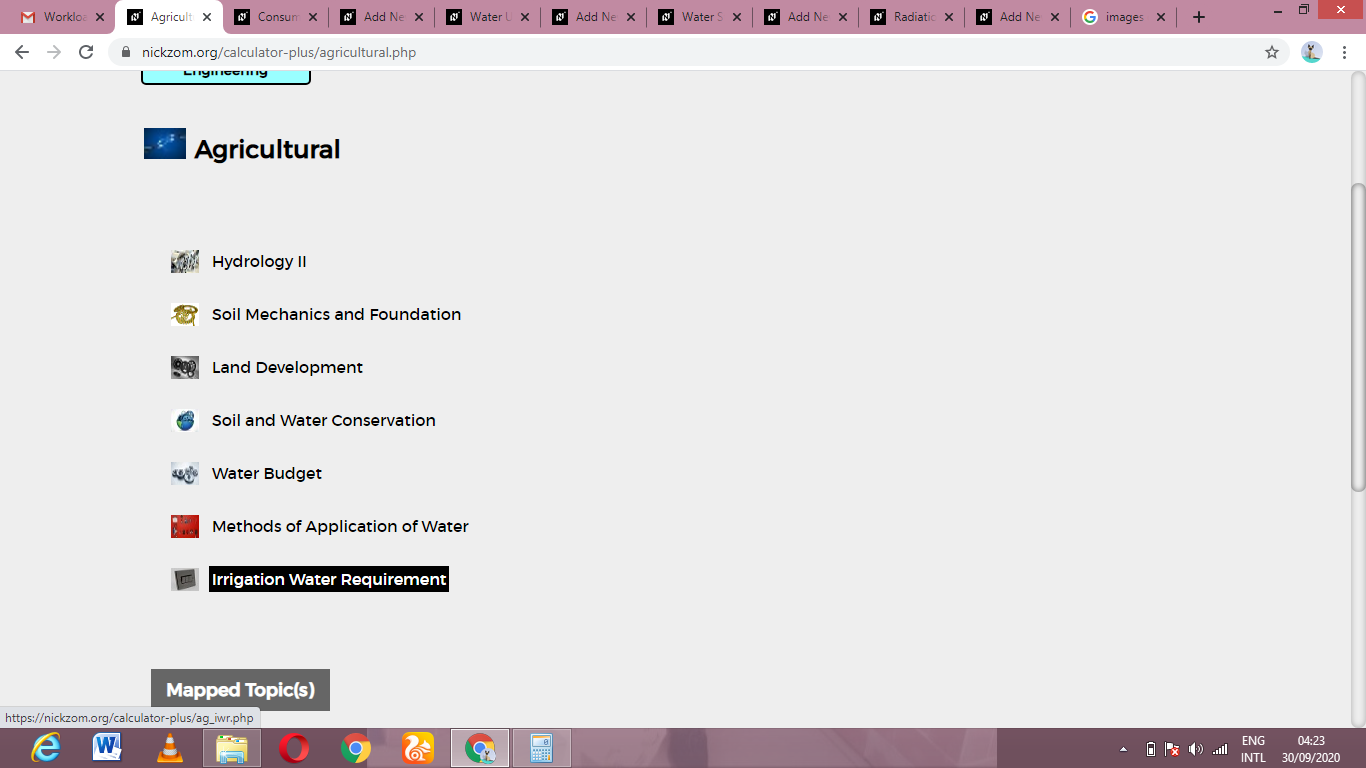Now, Click on Turbine Stiffness under Irrigation Water Requirement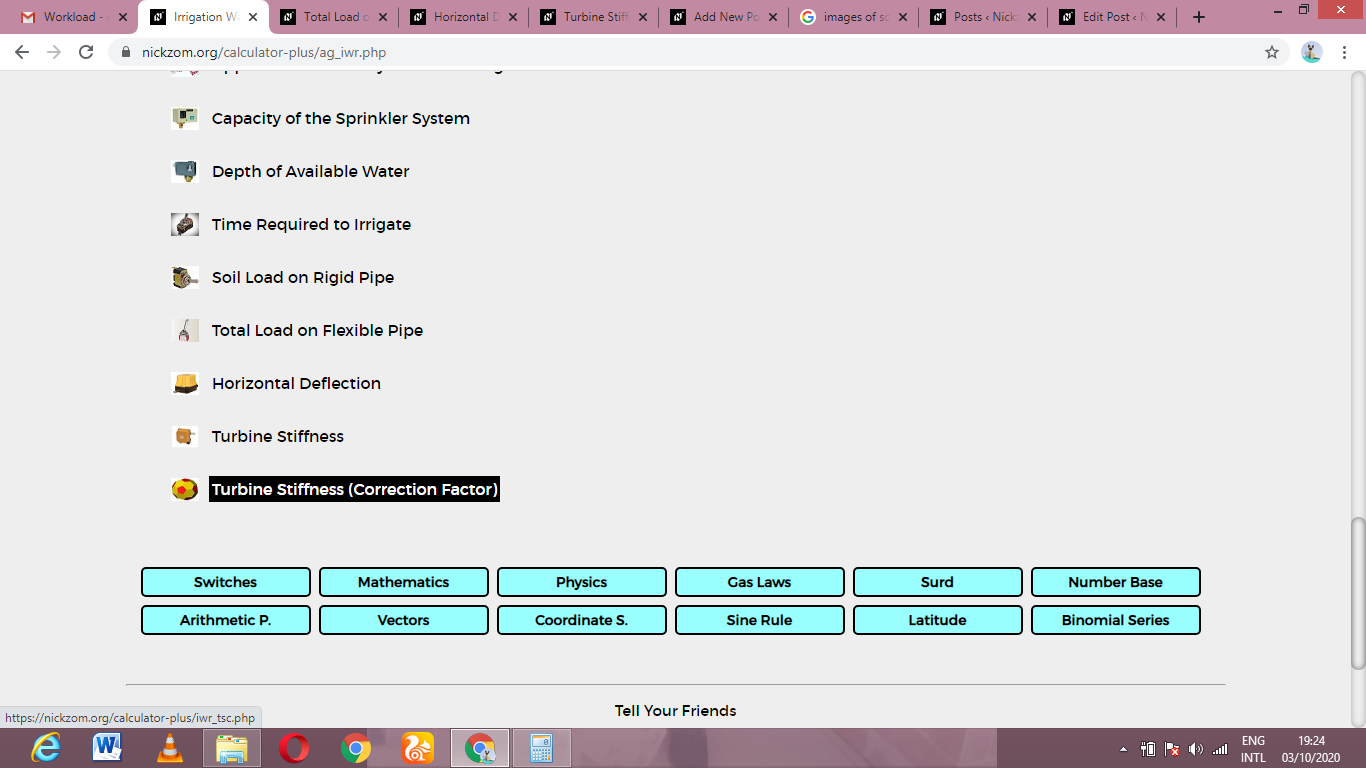The screenshot below displays the page or activity to enter your values, to get the answer for the turbine stiffness according to the respective parameters which is the Correction Factor for Radius of Curvature with Deflection (c) and Parallel Plate Stiffness for Tubing (σp).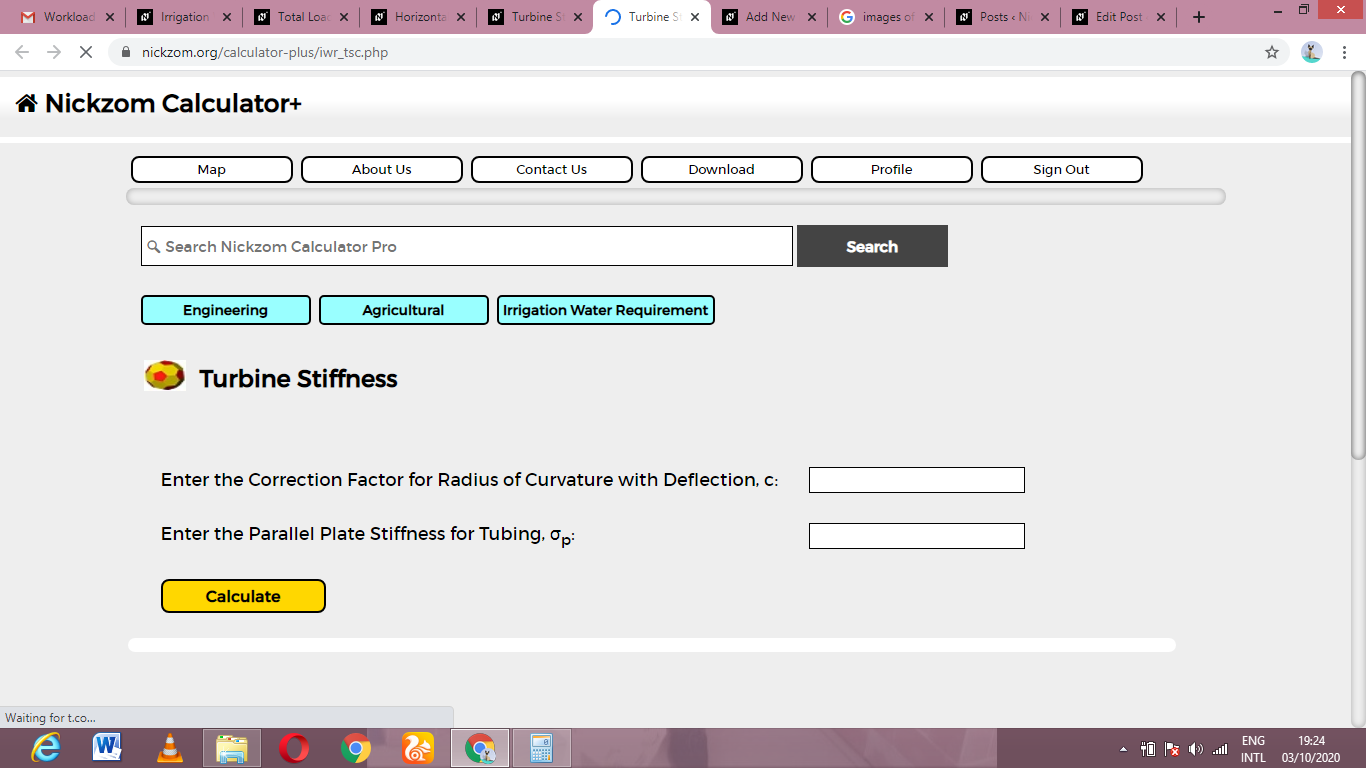Now, enter the values appropriately and accordingly for the parameters as required by the Correction Factor for Radius of Curvature with Deflection (c) is 15 and Parallel Plate Stiffness for Tubing (σp) is 3.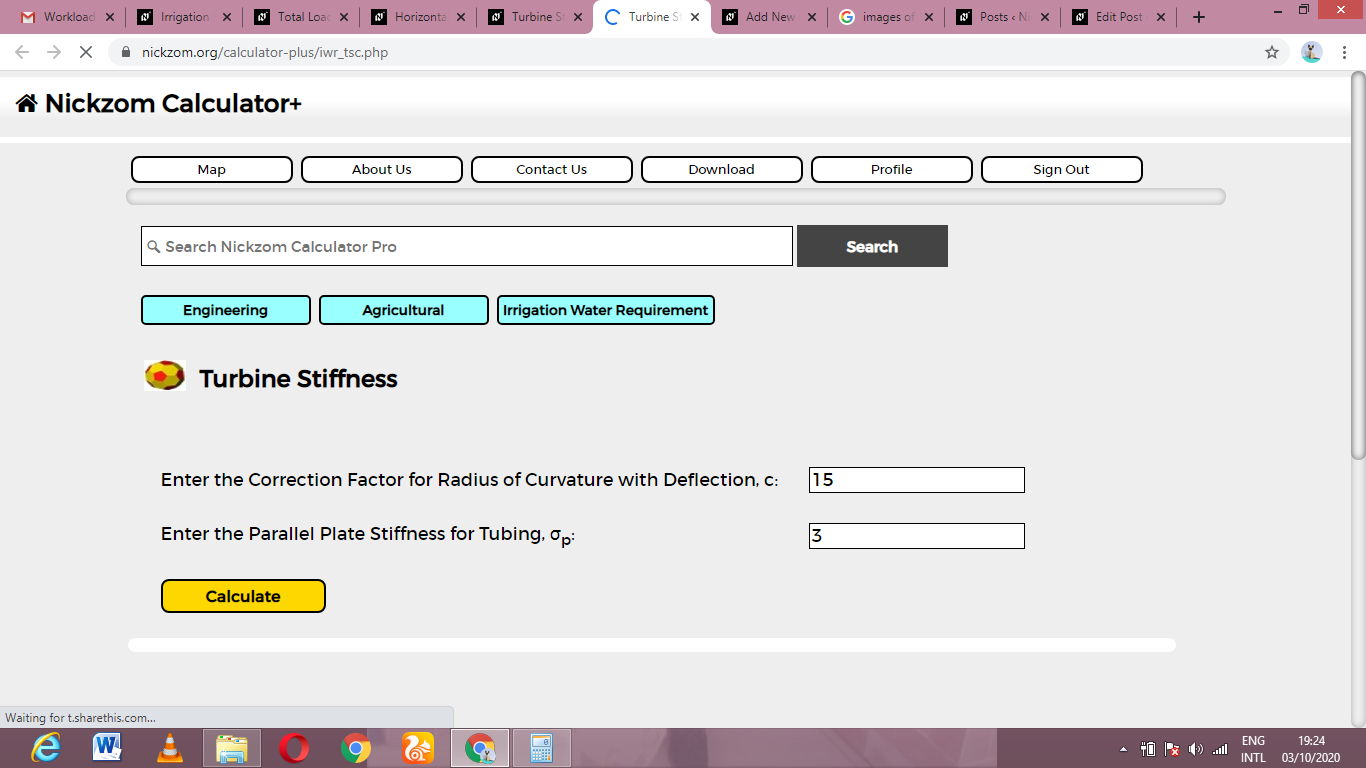Finally, Click on Calculate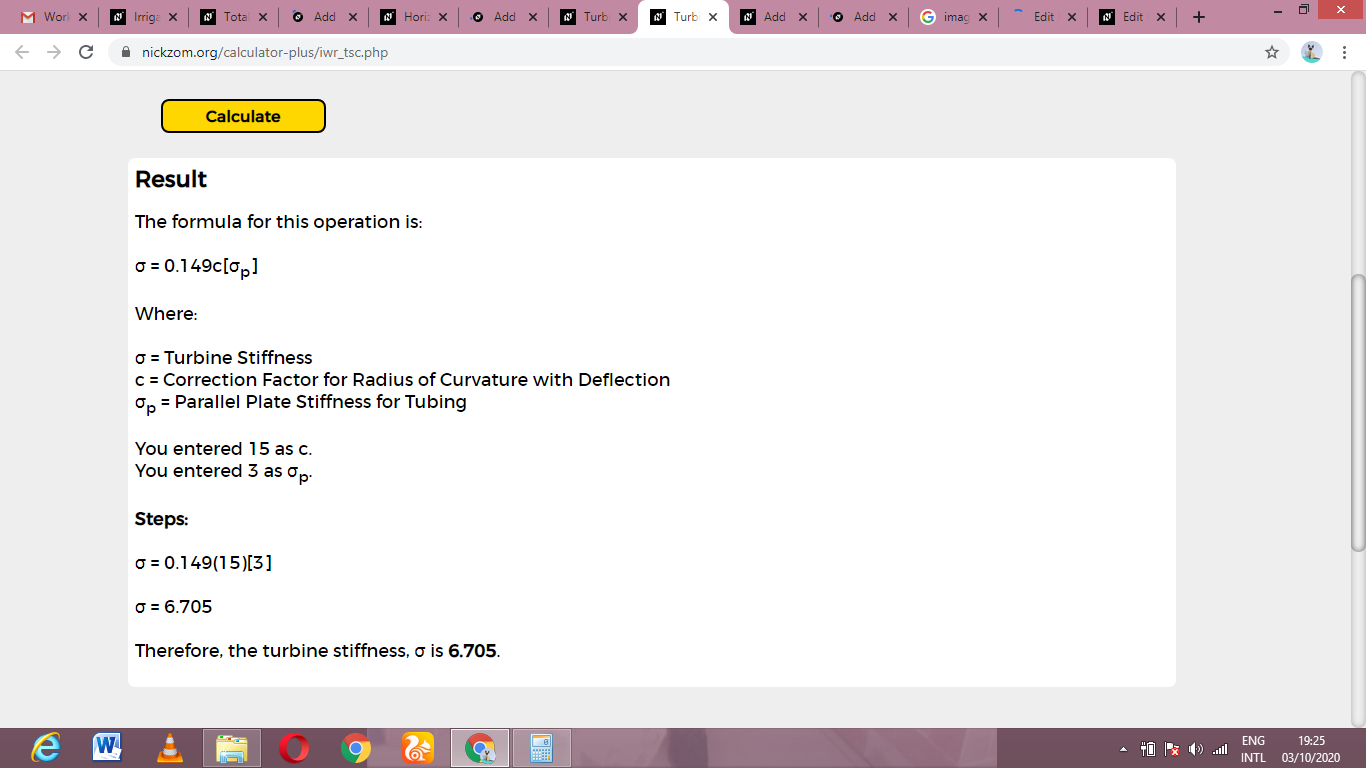As you can see from the screenshot above, Nickzom Calculator– The Calculator Encyclopedia solves for the turbine stiffness and presents the formula, workings and steps too.